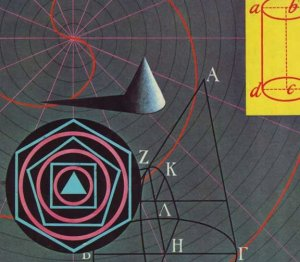A# mathematics"Pure mathematics consists entirely of assertions to the effect that if such and such a proposition is true of anything, then such and such another proposition is true of that thing. It is essential not to discuss whether the first proposition is really true, and not to mention what the anything is of which it is supposed to be true ... Thus mathematics may be defined as the subject in which we never know what we are talking about, nor whether what we are saying is true."
—Bertrand Russell

Mathematics is the study of patterns of quantity, structure, change, and space. The word 'mathematics' comes from the Greek mathema for 'knowledge' or 'that which is learned.' Historically, it grew out of the need to do calculations in commerce, to measure land, and to predict astronomical events. These three needs can be roughly related to the broad subdivision of mathematics into the study of structure, space, and change.

The study of structure starts with numbers – initially the familiar natural numbers and integers. The rules governing arithmetical operations are dealt with in elementary algebra, and the deeper properties of whole numbers are studied in number theory. The investigation of methods to solve equations leads to the field of abstract algebra, which, among other things, studies rings and fields, structures that generalize the properties possessed by the familiar numbers. The physically important concept of vector, generalized to vector spaces and studied in linear algebra, belongs to the two branches of structure and space.

The study of space originates with geometry, first the Euclidean geometry and trigonometry of everyday three-dimensional space, but later also generalized to non-Euclidean geometry, which plays a central role in general relativity theory. The modern fields of differential geometry and algebraic geometry generalize geometry in different directions: differential geometry emphasizes the concepts of coordinate system, smoothness, and direction, while in algebraic geometry geometrical objects arise as sets of solutions to polynomial equations.

Group theory investigates the concept of symmetry abstractly and provides a link between the studies of space and structure. Topology connects the study of space and the study of change by focusing on the concept of continuity. Understanding and describing change in measurable quantities is the common theme of the natural sciences, and calculus was developed as an invaluable tool for doing this. The central concept used to describe a changing variable is that of a function. Many problems lead quite naturally to relations between a quantity and its rate of change, and the methods to solve these are studied in the field of differential equations. The numbers used to represent continuous quantities are the real numbers, and the detailed study of their properties and the properties of real-valued functions is known as real analysis. For several reasons, it is convenient to generalize to the complex numbers, which are studied in complex analysis. Functional analysis focuses attention on (typically infinite-dimensional) spaces of functions, laying the groundwork for quantum mechanics among many other things. In order to clarify and investigate the foundations of mathematics, the fields of set theory, mathematical logic, and model theory were developed.

Since the time of the ancient Greeks, thought has been given to the ultimate nature of mathematics. What is its role and status in reality? Most crucially, is it invented or discovered? Leopold Kronecker was on the side of invention: "God made the integers; all the rest is the work of Man." Charles Hermite, by contrast, was clearly a Platonist: "There exists ... an entire world, which is the totality of mathematical truths, to which we have access only with our mind, just as a world of physical reality exists, the one like the other independent of ourselves, both of divine creation." The German physicist Heinrich Hertz went even further: "One cannot escape the feeling that these mathematical formulas have an independent existence and an intelligence of their own, that they are wiser that we are, wiser even than their discoverers, that we get more out of them than was originally put into them." G. H. Hardy summed up what many mathematicians today have a tendency to believe:1 " ... that mathematical reality lies outside us, that our function is to discover or observe it, and that the theorems which we prove, and which we describe grandiloquently as our 'creations,' are simply the notes of our observations." In other passage, he made this point more precisely: "317 is a prime, not because we think so, or because our minds are shaped in one way rather than another, but because it is so, because mathematical reality is built that way." We are struck by how well mathematics describes the behavior of the world in which we live. The universe, in fact, appears to have a deep mathematical infrastructure. Martin Gardner makes the bold claim that: "Mathematics is not only real, but it is the only reality. [The] ... entire universe is made of matter... And matter is made of particles... Now what are the particles made out of? They're not made out of anything. The only thing you can say about the reality of an electron is to cite its mathematical properties. So there's a sense in which matter has completely dissolved and what is left is just a mathematical structure."

### Reference

1. Hardy, G. H. A Mathematician's Apology. London: Cambridge University Press, 1941.